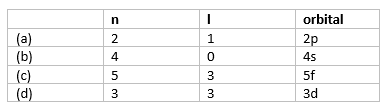# Quantum Numbers

## Quantum Numbers

A set of four quantum numbers which specify the energy, size, shape and orientation of an orbital about all the electrons present in an atom is called Quantum Numbers. To specify an orbital only three quantum numbers are required while to specify an electron all four quantum numbers are required.

Four quantum number are
$n$, $l$, $m_l$, $m_s$

### Principle quantum number ($n$):

• This quantum number tells energy level or shell to which electron belong
n = 1, 2, 3,…. (i.e. K,M,...)
• This also gives information about average distance of electron from nucleus and Energy of e- in hydrogen & hydrogen like atoms((He+, Li2+, .... etc.)
• Max number of electron present in a shell is given by 2n2
• Number of orbital in a shell is given by n2
 n 1 2 3 4 No of Orbitals(n2) 1 4 9 16 Max number of electron (2n2) 2 8 18 32

### Azimuthal/ subsidiary/ Orbital angular quantum number(l)

1. This gives us information about:
1. No. of sub – shell
2. angular momentum of electron
3. Shapes of various orbitals
4. energy of orbitals in multi-electron atoms along with principal quantum number
The number of orbitals in a subshell = 2l + 1. For a given value of n, l can have n values ranging from 0 to n-1. Total number of subshells in a particular shell is equal to the value of n.

 Subshell s p d f g Value of ‘l’ 0 1 2 3 4 Number of orbitals 1 3 5 7 9

Subshell Notation
 n l Subshell notation 1 0 1s 2 0 2s 2 1 2p 3 0 3s 3 1 3p 3 2 3d 4 0 4s 4 1 4p 4 2 4d 4 3 4f

### Magnetic Quantum number (ml )

It gives information about the No. of preferred orientations of electrons in sub – shell. Each orientation corresponds to orbital
Therefore   ml gives no. of orbitals in a sub shell
For any sub-shell (defined by ‘l’ value) 2l+1 values of ml are possible.
 L ml 0 0 (1 s orbital) 1 -1,0,1 (3 p orbital) 2 -2, -1,0,1,2 ( 5 d orbital) 3 -3,-2,-1,0,1,2,3 ( 7 f orbital) 4 -4,-3,-2,-1,0,1,2,3,4  (9 g orbitals)

### Electron Spin quantum number (ms )

It refers to orientation of the spin of the electron. It can have two values +1/2 and -1/2. +1/2 identifies the clockwise spin and -1/2 identifies the anti- clockwise spin.

Question 1
(a) How many subshell are possible with n = 4
(b) How many e- will be present in subshell having ;ms = -1/2 for n = 4.
Solution
For n = 4
l = 0 to (n – 1)
= 0, 1, 2, 3
l = 0      ml = -l to + 1 =0
l = 1      ml = -l to + l =-1, 0, 1
l = 2      ml = -l to + l = -2, -1, 0, 1, 2
l=3        ml = -l to + l = -3, -2, -1, 0, 1, 2, 3
For ms =-1/2 there will be 16 electrons

Question 2
Which of the following set of quantum no. is impossible?
a) n = 3, l = 2, m = -2, s = +1/2
b) n = 4, l = 0, m = 0, s = +1/2
c) n = 3, l = 2, m = -3, s = +1/2
d) n = 5, l = 3, m = 0, s = +1/2
Solution
n = 3
l = 0 to (n – 1)
= 0 to  2
0, 1, 2
s, p, d
m = -l to + l
l = 0      ml = -l to + 1 =0
l = 1      ml = -l to + l =-1, 0, 1
l = 2      ml = -l to + l = -2, -1, 0, 1, 2

Question 3
What is the total number of orbitals associated with the principal quantum number n = 3 ?
Solution
For n = 3, the possible values of l are 0, 1 and 2.
Thus there is one 3s orbital (n = 3, l = 0 and ml = 0)
There are three 3p orbitals (n = 3, l = 1 and ml = -1, 0, +1)
There are five 3d orbitals (n = 3, l = 2 and ml = -2, -1, 0, +1+, +2).
Therefore, the total number of orbitals is 1+3+5 = 9
The same value can also be obtained by using the relation; number of orbitals
= $n^2$, i.e. 32 = 9.

Question 4
Using s, p, d, f notations, describe the orbital with the following quantum numbers (a) n = 2, l = 1
(b) n = 4, l = 0
(c) n = 5,l = 3
(d) n = 3, l = 2
Solution